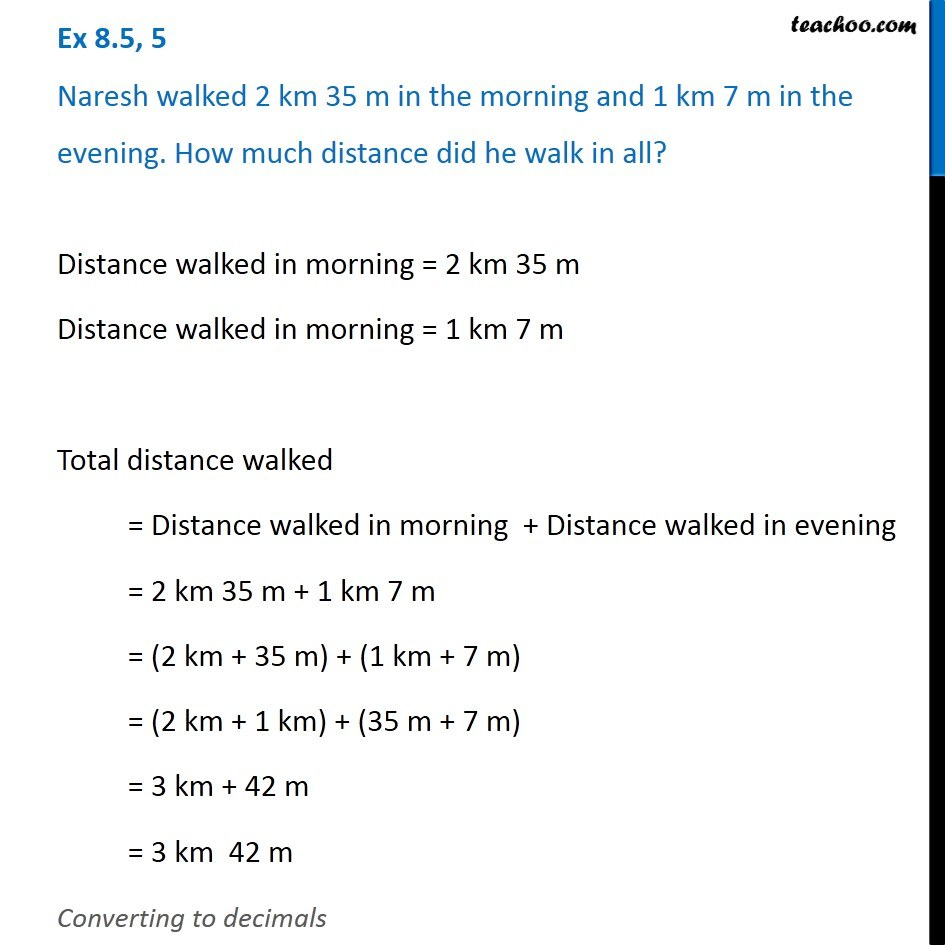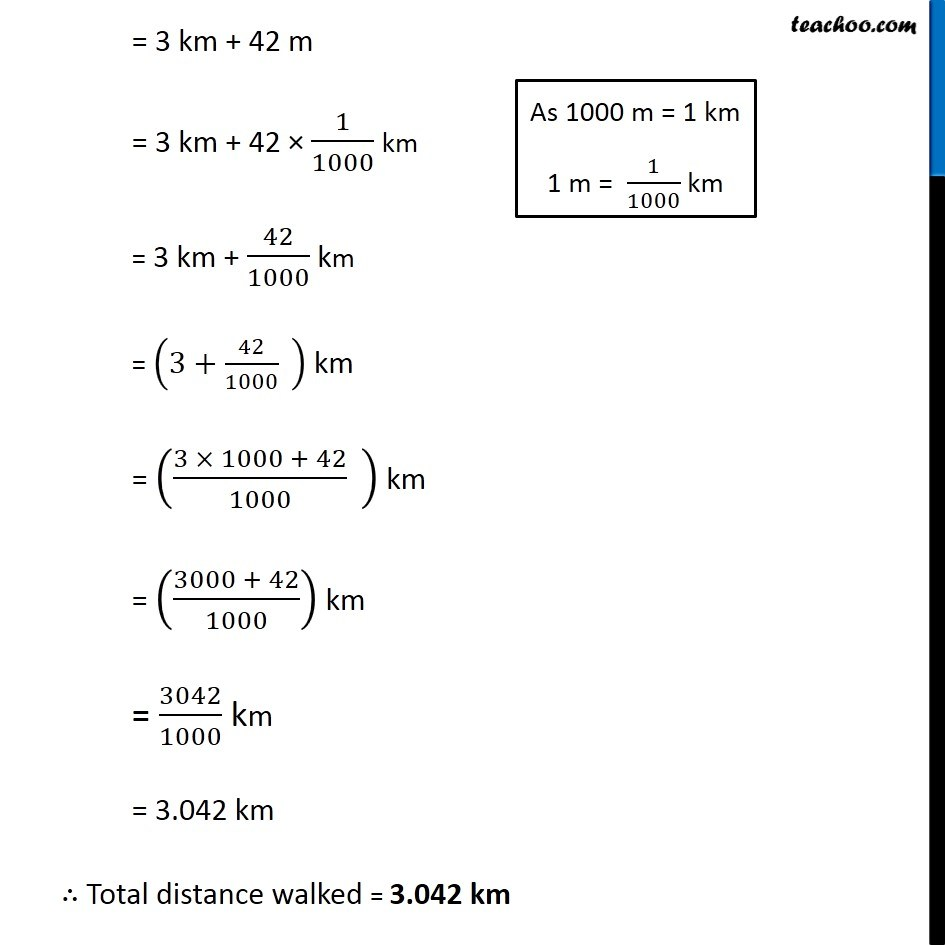Addition of decimal numbers - Statement questions

Chapter 8 Class 6 Decimals
Concept wiseLearn in your speed, with individual attention - Teachoo Maths 1-on-1 Class

### Transcript

Ex 8.3, 5 Naresh walked 2 km 35 m in the morning and 1 km 7 m in the evening. How much distance did he walk in all?Distance walked in morning = 2 km 35 m Distance walked in morning = 1 km 7 m Total distance walked = Distance walked in morning + Distance walked in evening = 2 km 35 m + 1 km 7 m = (2 km + 35 m) + (1 km + 7 m) = (2 km + 1 km) + (35 m + 7 m) = 3 km + 42 m = 3 km 42 m Converting to decimals = 3 km + 42 m = 3 km + 42 × 1/1000 km = 3 km + 42/1000 km = (3+42/1000 " " ) km = ((3 × 1000 + 42)/1000 " " ) km = ((3000 + 42)/1000) km = 3042/1000 km = 3.042 km ∴ Total distance walked = 3.042 km